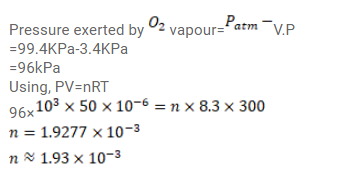Deepak Scored 45->99%ile with Bounce Back Crack Course. You can do it too!

# Use R=8.3 J/mol-K wherever required. 50 cc of oxygen is collected in an inverted gas jar over water.

Question:

Use $\mathrm{R}=8.3 \mathrm{~J} / \mathrm{mol}-\mathrm{K}$ wherever required.

$50 \mathrm{cc}$ of oxygen is collected in an inverted gas jar over water. The atmospheric pressure is $99.4 \mathrm{kPa}$ and the room temperature is $27^{\circ} \mathrm{C}$. The water level in the jar is same as the level outside. The saturation vapour pressure at $27^{\circ} \mathrm{C}$ is $3.4 \mathrm{kPa}$. Calculate the number of moles of oxygen collected in the jar.

Solution: